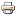Astronomy with SalsaJHow to weigh a galaxy

Determine the mass of a galaxy and discover dark matter!

This activity measures the mass of a spiral galaxy, viewed edge-on, using the same procedure employed by astronomers. It is surprising how just a few measurements and the knowledge of a few fundamental laws of physics make it possible to weigh the largest and most distant objects in the Universe, despite the impossibility of carrying out direct measurements and the fact that the only information available to us derive from a few photons which have travelled for tens and tens of millions of years. What is even more surprising is the fact that the simple measurement proposed here makes it possible to obtain experimental evidence for the well-known dark matter.Spiral galaxies contain a large amount of gas; the gas emits a spectrum of lines; if the galaxy is viewed edge-on (and not directly facing the observer, not perpendicular to the line of sight) and given that it rotates on its own axis, on one side the gas goes away from us and on the other, with respect to the centre, it draws closer to us (cf. figure). The lines emitted by a gas which moves with respect to the observer undergo the so-called Doppler effect, which shifts the observed frequency.The measurement we shall make exploits the fact that the Doppler Shift is proportional to the velocity at which the gas moves away or draws nearer to us. We therefore obtain the velocity v of the gas as a function of its distance r from the centre of the galaxy. The plot of the velocity v of each point of the galaxy as a function of the centre r is known as the rotation curve.

The velocity (assuming that the galaxy is in equilibrium and is subject to the Newtonian Law of universal gravity) is in turn proportional to the mass enclosed in the radius r.

This method is used by astronomers to calculate the mass of a large number of galaxies and is extremely important because it has made it possible to carry out systematic recording of an unexpected profile of the rotation curves, which is only explicable if it is assumed that the mass within the radius r far away from the galactic centre is much greater than the visible one, which is in other words the mass which emits radiation. This is better known as the famous problem of the dark matter, which today poses one of the most fascinating challenges to modern physics.

part 1 (data reduction) how_to_weight_a_galaxy_1

part 2 (calibration and measurement) how_to_weight_a_galaxy_2

IMAGES FOR MEASURING THE MASS OF  NGC7083 GALAXY

reduced spectra of the galaxy ngc7083 (you have to unzip the file)  - ngc7083_rid

calibration lamp image lamp_1